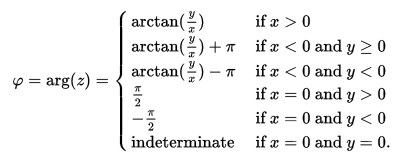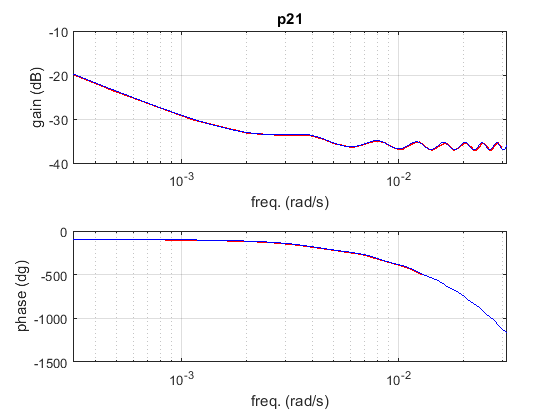# Bode plot of the Saint-Venant equations¶

This function is implemented based on Litrico, Xavier, and Vincent Fromion. “Frequency modeling of open-channel flow.” Journal of Hydraulic Engineering 130.8 (2004): 806-815.

## How to use¶

It is a Matlab function, that can be called with the following code:

```[freq, p21_mag, p21_phase_corr, p22_mag, p22_phase_corr, p11_mag, p11_phase_corr, p12_mag, p12_phase_corr ]=...
Bode_fun(m, B, Sb, n,q, x, Y0, min_frequency, max_frequency, TimePoints,SpacePoints)
```

where n is the Manning’s coefficient, q is the discharge, B is the bottom width, Sb is the bottom slope, Y0 is the downstream water depth and L is the length of the channel. The min_frequency and max_frequency are the exponents of 10. If zero is given to any of these quantities, then the minimun/maximum is taken 10 times less/more than the resonance frequency.

The TimePoints are the number of discretisation points in time. If zero is given, a default value of 1000 is used. Space points are the number of discretisation points in space. If zero is given, a default value of space step of 5m is used.

The first output is the frequency in rad/s. The gain values are output in decibels and the phase values in degrees.

## Implementation¶

Here some more comments should come…

For the calculation of the phase the atan2 function is used, giving the following results:After this operation in some cases 360 degrees are added in order to avoid big jumps. The necessity of adding it or subtracting it depends on the scale. This can be changed by changing the variable threshold_difference.

## Testing¶

The results are compared to the plots in the book Litrico, Xavier, and Vincent Fromion. Modeling and control of hydrosystems. Springer Science & Business Media, 2009. (page 49., Ex. 3.1 for p11 and p21 and p. 69, Ex 3.7 for p21 and p22) Red colour is the literature, blue colour is the written function.A small note for the tests: for the p11 case the height of the peaks depends on the resolution. However, in practice they are abscissas because the channel is horizontal and frictionless, thus theoretically a there is no force to dissipate the wave.